# Go Math Grade 4 Answer Key Homework Practice FL Chapter 9 Relate Fractions and Decimals

Go Math Grade 4 Answer Key Homework Practice FL Chapter 9 Relate Fractions and Decimals are provided here in pdf format for easy access. In HMH Go Math Answer Key Grade 4, students can find all chapters questions and solutions such as Relate Tenths and Decimals, Hundredths and Decimals, Equivalent Fractions, and Decimals, Relate Fractions, Decimals, and Money, Add Fractional Parts of 10 and 100, and many more. To help you out, we have complied Go Math Grade 4 Solution Key Homework Practice FL Chapter 9 Relate Fractions and Decimals for all chapters in a better way.

## Go Math Grade 4 Answer Key Homework Practice FL Chapter 9 Relate Fractions and Decimals

Browse all 9th chapter topics from Go Math Grade 4 Solution Key easily and learn the basics for further studies. It will be tough to understand the Chapter 9 Fractions and decimals concepts without a lot of practice. So, we have given the best preparation resource on this page ie., Go Math Grade 4 Answer Key Homework Practice FL Chapter 9 Relate Fractions and Decimals pdf. Download the Go Math HMH 4th Grade Answer Key of chapter 9 homework practice FL and learn the concepts properly to improve math skills.

Lesson: 1 – Relate Tenths and Decimals

Lesson: 2 – Relate Hundredths and Decimals

Lesson: 3 – Equivalent Fractions and Decimals

Lesson: 4 – Relate Fractions, Decimals, and Money

Lesson: 5 – Problem Solving Money

Lesson: 6 – Add Fractional Parts of 10 and 100

Lesson: 7 – Compare Decimals

Lesson: 8

### Common Core – Relate Fractions and Decimals – Page No. 171

Relate Tenths and Decimals

Write the fraction or mixed number and the decimal shown by the model.

Question 1.Question 2.Type below:
_________
1 2/10

Explanation:
The model is divided into 10 equal parts. Each part represents one-tenth.
1 2/10 is 1 whole and 2 tenths.

Question 3.Type below:
_________

2 3/10 = 2.3

Explanation: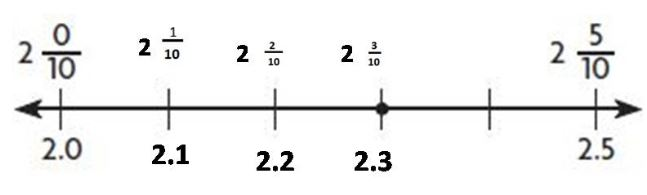By seeing the above number line we can say that the decimal is 2.3

Question 4.Type below:
_________

4810 = 4.8

Explanation: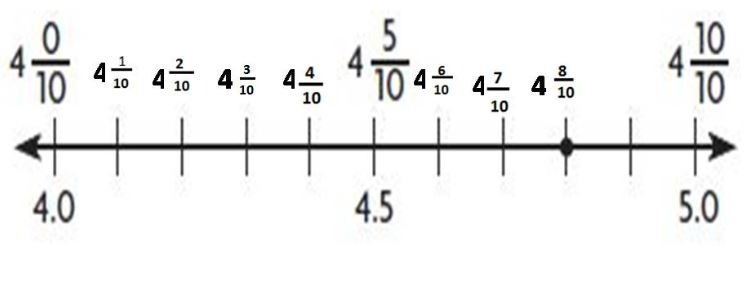Write the fraction or mixed number as a decimal.

Question 5.
$$\frac{4}{10}$$
_____

0.4

Explanation:
Write down 4 with the decimal point 1 space from the right (because 10 has 1 zero)
0.4
The decimal form for the fraction $$\frac{4}{10}$$ is 0.4

Question 6.
3 $$\frac{1}{10}$$
_____

3.1

Explanation:
Multiply 3 x 10 = 30.
Add 30 + 1 = 31.
So, 31/10.
Write down 31 with the decimal point 1 space from the right (because 10 has 1 zero)
3.1

Question 7.
$$\frac{7}{10}$$
_____

0.7

Explanation:
Write down 7 with the decimal point 1 space from the right (because 10 has 1 zero)
0.7
The decimal form for the fraction $$\frac{7}{10}$$ is 0.7

Question 8.
6 $$\frac{5}{10}$$
_____

6.5

Explanation:
Multiply 6 x 10 = 60.
Add 60 + 5 = 65.
So, 65/10.
Write down 35 with the decimal point 1 space from the right (because 10 has 1 zero)
6.5

Question 9.
$$\frac{9}{10}$$
_____

0.9

Explanation:
Write down 9 with the decimal point 1 space from the right (because 10 has 1 zero)
0.9
The decimal form for the fraction $$\frac{9}{10}$$ is 0.9

Problem Solving

Question 10.
There are 10 sports balls in the equipment closet. Three are kickballs. Write the portion of the balls that are kickballs as a fraction, as a decimal, and in word form.
Type below:
_________

3/10 = 0.3 = three tenths

Explanation:
Given,
There are 10 sports balls in the equipment closet.
Three are kickballs.
So, 3/10 kickballs are available.

Question 11.
Peyton has 2 pizzas. Each pizza is cut into 10 equal slices. She and her friends eat 14 slices. What part of the pizzas did they eat? Write your answer as a decimal.
_________

1.4 pizzas

Explanation:
Peyton has 2 pizzas. Each pizza is cut into 10 equal slices.
So, total number of slices = 2 x 10 = 20.
She and her friends eat 14 slices.
So, they ate 1 whole pizza and 4 parts out of 10 slices in the second pizza.
1 4/10 = 14/10 = 1.4 pizzas.
Therefore the decimal form of the part of the pizzas they eat is 1.4 pizzas.

### Common Core – Relate Fractions and Decimals – Page No. 172

Lesson Check

Question 1.
Valerie has 10 CDs in her music case. Seven of the CDs are pop music CDs. What is this amount written as a decimal?
Options:
a. 70.0
b. 7.0
c. 0.7
d. 0.07

c. 0.7

Explanation:
Valerie has 10 CDs in her music case. Seven of the CDs are pop music CDs.
Seven CDs out of 10 CDs = 7/10 =0.7
Thus the correct answer is option c.

Question 2.
Which decimal amount is modeled below?Options:
a. 140.0
b. 14.0
c. 1.4
d. 0.14

c. 1.4

Explanation:
1 4/10
Multiply 10 x 1 = 10.
Add 10 + 4 = 14.
So, 14/10 = 1.4.
Thus the correct answer is option c.

Spiral Review

Question 3.
Which number is a factor of 13?
Options:
a. 1
b. 3
c. 4
d. 7

a. 1

Explanation:
13 has 1 and 13 as its factors.
Thus the correct answer is option a.

Question 4.
An art gallery has 18 paintings and 4 photographs displayed in equal rows on a wall, with the same number of each type of art in each row. Which of the following could be the number of rows?
Options:
a. 2 rows
b. 3 rows
c. 4 rows
d. 6 rows

a. 2 rows

Explanation:
An art gallery has 18 paintings and 4 photographs displayed in equal rows on a wall, with the same number of each type of art in each row. So, 18 paintings and 4 photographs need to be divided into equal parts.
18/2 = 9; 4/2 = 2.
2 rows can be possible with 9 pictures and 2 pictures in each row.
Thus the correct answer is option a.

Question 5.
How do you write the mixed number shown as a fraction greater than 1?Options:
a. $$\frac{32}{5}$$
b. $$\frac{14}{4}$$
c. $$\frac{6}{4}$$
d. $$\frac{4}{4}$$

b. 14/4
Explanation:
324 = 14/4. 14 divided by 4 is equal to 3 with a remainder of 2. The 3 is greater than 1. So, 14/4 > 1.
Thus the correct answer is option b.

Question 6.
Which of the following models has an amount shaded that is equivalent to the fraction $$\frac{1}{5}$$?
Options:
a.b.c.d.c.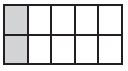Explanation:
a. 2/3
b. 5/10 = 1/2
c. 2/10 = 1/5
d. 1/10
Thus the correct answer is option c.

### Common Core – Relate Fractions and Decimals – Page No. 173

Relate Hundredths and Decimals

Write the fraction or mixed number and the decimal shown by the model.

Question 1.Question 2.Type below:
_________

29/100 = 0.29

Explanation:
0.20 names the same amount as 20/100.
So, the given point is at 29/100 = 0.29

Question 3.Type below:
_________

1 54/100 = 1.54

Explanation:
From the given image, one model is one whole and another model 54 boxes shaded out of 100. So, the answer is 1 54/100 = 1.54

Question 4.Type below:
_________

4 62/100 = 4.62

Explanation:
4.60 names the same amount as 460100. So, the given point is at 4 62/100 = 4.62

Write the fraction or mixed number as a decimal.

Question 5.
$$\frac{37}{100}$$
_____

0.37

Explanation:
Write down 37 with the decimal point 2 spaces from the right (because 100 has 2 zeros). 0.37

Question 6.
8 $$\frac{11}{100}$$
_____

8.11

Explanation:
8 11/100 = 811/100
Write down 811 with the decimal point 2 spaces from the right (because 100 has 2 zeros). So, 8.11 is the answer.

Question 7.
$$\frac{98}{100}$$
_____

0.98

Explanation:
Write down 98 with the decimal point 2 spaces from the right (because 100 has 2 zeros). 0.98

Question 8.
25 $$\frac{50}{100}$$
_____

25.50

Explanation:
25 50/100 = 2550/100
Write down 2550 with the decimal point 2 spaces from the right (because 100 has 2 zeros). So, 25.50 is the answer.

Question 9.
$$\frac{6}{100}$$
_____

0.06

Explanation:
Write down 6 with the decimal point 2 spaces from the right (because 100 has 2 zeros). 0.06

Problem Solving

Question 10.
There are 100 pennies in a dollar. What fraction of a dollar is 61 pennies? Write it as a fraction, as a decimal, and in word form.
Type below:
_________

61/100 pennies = 0.61 = sixty-one hundredths

Explanation:
There are 100 pennies in a dollar. So, for 61 pennies, there are 61100 pennies = 0.61 = sixty-one hundredths.

Question 11.
Kylee has collected 100 souvenir thimbles from different places she has visited with her family. Twenty of the thimbles are carved from wood. Write the fraction of thimbles that are wooden as a decimal.
_________

It is easier to work with decimals then fractions because it is like adding whole numbers in a normal way.

### Common Core – Relate Fractions and Decimals – Page No. 174

Lesson Check

Question 1.
Which decimal represents the shaded section of the model below?Options:
a. 830.0
b. 83.0
c. 8.30
d. 0.83

d. 0.83

Explanation:
The model is divided into 100 equal parts. Each part represents one hundredth. 83 boxes are shaded out of 100.
So, the answer is 83/100 = 0.83
Thus the correct answer is option d.

Question 2.
There were 100 questions on the unit test. Alondra answered 97 of the questions correctly. What decimal represents the fraction of questions Alondra answered correctly?
Options:
a. 0.97
b. 9.70
c. 90.70
d. 970.0

a. 0.97

Explanation:
There were 100 questions on the unit test. Alondra answered 97 of the questions correctly. So, 97/100 questions answered correctly. = 0.97
Thus the correct answer is option a.

Spiral Review

Question 3.
Which is equivalent to $$\frac{7}{8}$$ ?
Options:
a. $$\frac{5}{8}+\frac{3}{8}$$
b. $$\frac{4}{8}+\frac{1}{8}+\frac{1}{8}$$
c. $$\frac{3}{8}+\frac{2}{8}+\frac{2}{8}$$
d. $$\frac{2}{8}+\frac{2}{8}+\frac{1}{8}+\frac{1}{8}$$

c. 3/8+2/8+2/8
Explanation:
c. 3/8+2/8+2/8 = 7/8
Thus the correct answer is option c.

Question 4.
What is $$\frac{9}{10}-\frac{6}{10}$$?Options:
a. $$\frac{1}{10}$$
b. $$\frac{3}{10}$$
c. $$\frac{4}{10}$$
d. $$\frac{6}{10}$$

b. 3/10
Explanation:
9/10−6/10. From 9 parts, 6 parts are removed. So, remaining parts are 3.
Thus the correct answer is option b.

Question 5.
Misha used 14 of a carton of 12 eggs to make an omelet. How many eggs did she use?
Options:
a. 2
b. 3
c. 4
d. 6

b. 3

Explanation:
Misha used 14 of a carton of 12 eggs to make an omelet. 14 x 12 = 3 eggs.
Thus the correct answer is option b.

Question 6.
Kurt used the rule add 4, subtract 1 to generate a pattern. The first term in his pattern is 5. Which number could be in Kurt’s pattern?
Options:
a. 4
b. 6
c. 10
d. 14

d. 14

Explanation:
Kurt used the rule add 4, subtract 1 to generate a pattern.
The first term in his pattern is 5.
The pattern numbers are 5, 8, 11, 14, 17, 20, etc.
Thus the correct answer is option d.

### Common Core – Relate Fractions and Decimals – Page No. 175

Equivalent Fractions and Decimals

Write the number as hundredths in fraction form and decimal form.

Question 1.
$$\frac{5}{10}$$
$$\frac{5}{10}$$ = $$\frac{5 \times 10}{10 \times 10}=\frac{50}{100}$$Think: 5 tenths is the same as 5 tenths and 0 hundredths. Write 0.50.

Question 2.
$$\frac{9}{10}$$
Type below:
_________

90/100; 0.90

Explanation:
9/10 = 9×10/10×10 = 90/100
9 tenths is the same as 9 tenths and 0 hundredths. Write 0.90

Question 3.
0.2
Type below:
_________

20100
0.20

Explanation:
2 tenths is the same as 2 tenths and 0 hundredths. Write 0.20.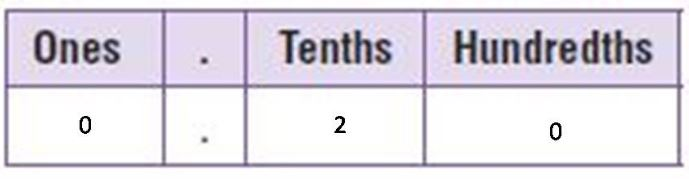2/10 = 2×10/10×10 = 20/100

Question 4.
0.8
Type below:
_________

80100 = 0.80

Explanation:
8 tenths is the same as 8 tenths and 0 hundredths. Write 0.80.8/10 = 8×10/10×10 = 80/100

Write the number as tenths in fraction form and decimal form.

Question 5.
$$\frac{40}{100}$$
Type below:
_________

4/10 = 0.4

Explanation:
10 is a common factor of the numerator and the denominator.
40/100 = 40÷10/100÷10 = 4/10
= 0.4

Question 6.
$$\frac{10}{100}$$
Type below:
_________

1/10 = 0.1

Explanation:
10 is a common factor of the numerator and the denominator.
10/100 = 10÷10/100÷10 = 1/10
= 0.1

Question 7.
0.60
Type below:
_________

6/10 = 0.6

Explanation:
0.60 is 60 hundredths.
60/100.
10 is a common factor of the numerator and the denominator.
60/100 = 60÷10/100÷10 = 6/10
= 0.6

Problem Solving

Question 8.
Billy walks $$\frac{6}{10}$$ mile to school each day. Write $$\frac{6}{10}$$ as hundredths in fraction form and in decimal form.
Type below:
_________

60100
0.60

Explanation:
Billy walks 6/10 mile to school each day.
6/10 = 6×10/10×10 = 60/100

Question 9.
Four states have names that begin with the letter A. This represents 0.08 of all the states. Write 0.08 as a fraction.
$$\frac{□}{□}$$

8/100
Explanation:
0.08 is 8 hundredths. So, the fraction is 8/100

### Common Core – Relate Fractions and Decimals – Page No. 176

Lesson Check

Question 1.
The fourth-grade students at Harvest School make up 0.3 of all students at the school. Which fraction is equivalent to 0.3?
Options:
a. $$\frac{3}{10}$$
b. $$\frac{30}{10}$$
c. $$\frac{3}{100}$$
d. $$\frac{33}{100}$$

a. 3/10
Explanation:
0.3 is same as the 3 tenths.
Thus the correct answer is option a.

Question 2.
Kyle and his brother have a marble set. Of the marbles, 12 are blue. This represents $$\frac{50}{100}$$ of all the marbles. Which decimal is equivalent to $$\frac{50}{100}$$?
Options:
a. 50
b. 5.0
c. 0.50
d. 5,000

c. 0.50

Explanation:

Write down 50 with the decimal point 2 spaces from the right (because 100 has 2 zeros).
Thus the correct answer is option c.

Spiral Review

Question 3.
Jesse won his race by 3 $$\frac{45}{100}$$ seconds. What is this number written as a decimal?
Options:
a. 0.345
b. 3.45
c. 34.5
d. 345

b. 3.45

Explanation:
3 45/100 = 345/100. Write down 345 with the decimal point 2 spaces from the right (because 100 has 2 zeros). So, 3.45 is the answer.
Thus the correct answer is option b.

Question 4.
Marge cut 16 pieces of tape for mounting pictures on poster board. Each piece of tape was $$\frac{3}{8}$$ inch long. How much tape did Marge use?
Options:
a. 2 inches
b. 4 inches
c. 5 inches
d. 6 inches

d. 6 inches

Explanation:
3/8 x 16 = 6 inches
Thus the correct answer is option d.

Question 5.
Of Katie’s pattern blocks, $$\frac{9}{12}$$ are triangles. What is $$\frac{9}{12}$$ in simplest form?
Options:
a. $$\frac{1}{4}$$
b. $$\frac{2}{3}$$
c. $$\frac{3}{4}$$
d. $$\frac{9}{12}$$

c. 3/4
Explanation:
9/12 is divided by 3. So, 3/4 is the answer.
Thus the correct answer is option c.

Question 6.
A number pattern has 75 as its first term. The rule for the pattern is subtract 6. What is the sixth term?
Options:
a. 39
b. 45
c. 51
d. 69

b. 45

Explanation:
75 is the first term.
75 – 6 =69
69 – 6 = 63
63 – 6 = 57
57 – 6 = 51
51 – 6 = 45.
The sixth term is 45.
Thus the correct answer is option b.

### Common Core – Relate Fractions and Decimals – Page No. 177

Relate Fractions, Decimals, and Money

Write the total money amount. Then write the amount as a fraction or a mixed number and as a decimal in terms of dollars.

Question 1.$0.18 = 18/100 = 0.18 Explanation: Given that 3 Pennies + 3 Nickels = 3/100 + 15/100 = 18/100 Question 2.Type below: _________ Answer:$0.56 = 56/100 = 0.56

Explanation:
Given that 1 Quarter + 3 dime + 1 Pennies = 25/100 + 30/100 + 1/100 = 56/100

Write as a money amount and as a decimal in terms of dollars.

Question 3.
$$\frac{25}{100}$$
Dollars: _____ Decimal: _____

Dollars: 1 quarter = $0.25; Decimal: 0.25 Explanation: 25 our of 100 dollars = 1 quarter. So, 25/100 = 0.25 Question 4. $$\frac{79}{100}$$ Dollars: _____ Decimal: _____ Answer: amount:$0.79 decimal: 0.79 of a dollar

Explanation:
79/100 = 0.79

Question 5.
$$\frac{31}{100}$$
Dollars: _____ Decimal: _____

amount: $0.31 decimal: 0.31 of a dollar Explanation: 31/100 = 0.31 Question 6. $$\frac{8}{100}$$ Dollars: _____ Decimal: _____ Answer: amount:$0.08 decimal: 0.08 of a dollar

Explanation:
81/00 = 0.08

Question 7.
$$\frac{42}{100}$$
Dollars: _____ Decimal: _____

amount: $0.42 decimal: 0.42 of a dollar Explanation: 42/100 = 0.42 Write the money amount as a fraction in terms of dollars. Question 8.$0.87
$$\frac{□}{□}$$

87/100 of a dollar

Explanation: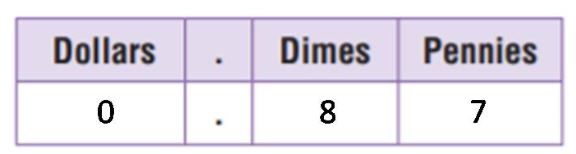$0.87 = 87 pennies There are 100 pennies in 1 dollar. So,$0.87 = 87/100 of a dollar.

Question 9.
$0.03 $$\frac{□}{□}$$ Answer: 3/100 Explanation: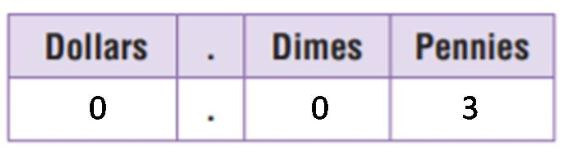$0.03 = 3 pennies
There are 100 pennies in 1 dollar.
So, $0.03 = 3/100. Question 10.$0.66
$$\frac{□}{□}$$

66/100

Explanation: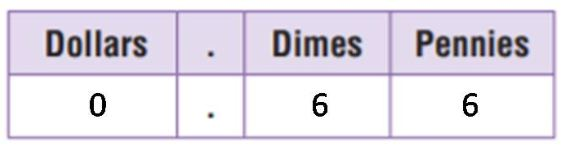$0.66 = 66 pennies There are 100 pennies in 1 dollar. So,$0.66 = 66/100.

Question 11.
$0.95 $$\frac{□}{□}$$ Answer: 95/100 Explanation: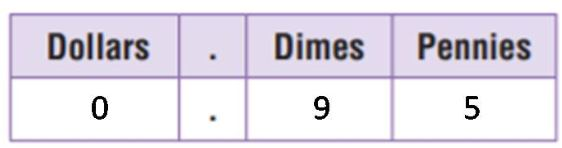$0.95 = 95 pennies
There are 100 pennies in 1 dollar.
So, $0.95 = 95/100. Question 12.$1.00
$$\frac{□}{□}$$

100/100

Explanation: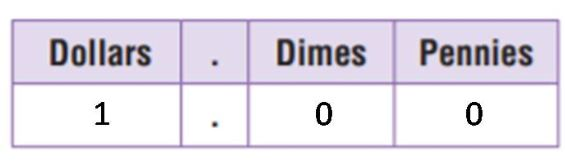$1.00 = 1 dollar There are 100 pennies in 1 dollar. So,$1.00 = 100/100.

Write the total money amount. Then write the amount as a fraction and as a decimal in terms of dollars.

Question 13.
2 quarters 2 dimes
Type below:
_________

money amount: $0.70; fraction: 70/100; decimal: 0.70 Explanation: Given that 2 quarters 2 dimes = (2 x 25/100) + (2 x 10/100) = 50/100 + 20/100 = 70/100 Question 14. 3 dimes 4 pennies Type below: _________ Answer: money amount:$0.34; fraction: 34/100; decimal: 0.34

Explanation:
Given that 3 dimes 4 pennies = (3 x 10/100) + (4 x 1/100) = 30/100 + 4/100 = 34/100

Question 15.
8 nickels 12 pennies
Type below:
_________

money amount: $0.57; fraction: 57/100; decimal: 0.57 Explanation: Given that 8 nickels 12 pennies = (8 x 5/100) + (12 x 1/100) = 45/100 + 12/100 = 57/100 Problem Solving Question 16. Kate has 1 dime, 4 nickels, and 8 pennies. Write Kate’s total amount as a fraction in terms of a dollar. $$\frac{□}{□}$$ Answer: fraction: 38/100 Explanation: Kate has 1 dime, 4 nickels, and 8 pennies. 10/100 + (4 x 5/100) + (8/100) = 10/100 + 20/100 + 8/100 = 38/100 Question 17. Nolan says he has $$\frac{75}{100}$$ of a dollar. If he only has 3 coins, what are the coins? __________ Answer: 3 quarters Explanation: 3 quarters = 25/100 + 25/100 + 25/100 = 75/100 ### Common Core – Relate Fractions and Decimals – Page No. 178 Lesson Check Question 1. Which of the following names the total money amount shown as a fraction in terms of a dollar?Options: a. $$\frac{43}{1}$$ b. $$\frac{43}{10}$$ c. $$\frac{43}{57}$$ d. $$\frac{43}{100}$$ Answer: d. 43/100 Explanation: Given that 1 quarter + 1 nickel + 1 dime + 3 pennies = 25/100 + 5/100 + 10/100 + 3/100 = 43/100 Thus the correct answer is option d. Question 2. Crystal has $$\frac{81}{100}$$ of a dollar. Which of the following could be the coins Crystal has? Options: a. 3 quarters, 1 dime, 1 penny b. 2 quarters, 6 nickels, 1 penny c. 2 quarters, 21 pennies d. 1 quarter, 4 dimes, 1 nickel, 1 penny Answer: b. 2 quarters, 6 nickels, 1 penny Explanation: 2 quarters, 6 nickels, 1 penny = (2 x 25/100) + (6 x 5/100) + 1/100 = 50/100 + 30/100 + 1/100 = 81/100 Thus the correct answer is option b. Spiral Review Question 3. Joel gives $$\frac{1}{3}$$ of his baseball cards to his sister. Which fraction is equivalent to $$\frac{1}{3}$$? Options: a. $$\frac{3}{5}$$ b. $$\frac{2}{6}$$ c. $$\frac{8}{9}$$ d. $$\frac{4}{10}$$ Answer: b. 2/6 Explanation: 2/6 is divided by 2. The remaining answer after the dividion is 1/3. Thus the correct answer is option b. Question 4. Penelope bakes pretzels. She salts $$\frac{3}{8}$$ of the pretzels. Which fraction is equivalent to $$\frac{3}{8}$$ ? Options: a. $$\frac{9}{24}$$ b. $$\frac{15}{20}$$ c. $$\frac{3}{16}$$ d. $$\frac{1}{5}$$ Answer: a. 9/24 Explanation: a. 9/24 is divided by 3. The remaining fraction after the division is 3/8. Thus the correct answer is option a. Question 5. Which decimal is shown by the model?Options: a. 10.0 b. 1.0 c. 0.1 d. 0.01 Answer: d. 0.01 Explanation: 1 box is shaded out of 100. So, the fraction is 1/100 = 0.01. Thus the correct answer is option d. Question 6. Mr. Guzman has 100 cows on his dairy farm. Of the cows, 57 are Holstein. What decimal represents the portion of cows that are Holstein? Options: a. 0.43 b. 0.57 c. 5.7 d. 57.0 Answer: b. 0.57 Explanation: Mr. Guzman has 100 cows on his dairy farm. Of the cows, 57 are Holstein. So, 57/100 Holstein cows are available. 57/100 = 0.57 Thus the correct answer is option b. ### Common Core – Relate Fractions and Decimals – Page No. 179 Problem Solving Money Use the act it out strategy to solve. Question 1. Carl wants to buy a bicycle bell that costs$4.50. Carl has saved $2.75 so far. How much more money does he need to buy the bell? Use 4$1 bills and 2 quarters to model $4.50. Remove bills and coins that have a value of$2.75. First, remove 2 $1 bills and 2 quarters. Next, exchange one$1 bill for 4 quarters and remove 1 quarter.
Count the amount that is left. So, Carl needs to save $1.75 more.Answer: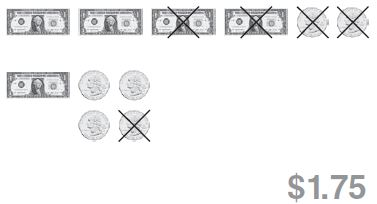Question 2. Together, Xavier, Yolanda, and Zachary have$4.44. If each person has the same amount, how much money does each person have?
$_________ Answer:$1.11

Explanation:
Together, Xavier, Yolanda, and Zachary have $4.44. If each person has the same amount,$4.44/4 = $1.11 Question 3. Marcus, Nan, and Olive each have$1.65 in their pockets. They decide to combine the money. How much money do they have altogether?
$_________ Answer:$4.95

Explanation:
Marcus, Nan, and Olive each have $1.65 in their pockets. They decide to combine the money. So,$1.65 + $1.65 +$1.65 = $4.95 Question 4. Jessie saves$6 each week. In how many weeks will she have saved at least $50? _________ weeks Answer: 9 weeks Explanation: Jessie saves$6 each week. To save $50,$50/$6 = 9 weeks (approximately) Question 5. Becca has$12 more than Cece. Dave has $3 less than Cece. Cece has$10. How much money do they have altogether?
$_________ Answer:$39

Explanation:
Cece has $10. Becca has$12 more than Cece = $10 +$12 = $22. Dave has$3 less than Cece = $10 –$3 = $7. All together =$10 + $22 +$7 = $39. ### Common Core – Relate Fractions and Decimals – Page No. 180 Lesson Check Question 1. Four friends earned$5.20 for washing a car. They shared the money equally. How much did each friend get?
Options:
a. $1.05 b.$1.30
c. $1.60 d.$20.80

b. $1.30 Explanation: Four friends earned$5.20 for washing a car. They shared the money equally.
$5.20/4 =$1.30
Thus the correct answer is option b.

Question 2.
Which represents the value of one $1 bill and 5 quarters? Options: a.$1.05
b. $1.25 c.$1.50
d. $2.25 Answer: d.$2.25

Explanation:
one $1 bill and 5 quarters. 5 quarters = 5 x 0.25 = 1.25.$1 + $1.25 =$2.25
Thus the correct answer is option d.

Spiral Review

Question 3.
Bethany has 9 pennies. What fraction of a dollar is this?
Options:
a. $$\frac{9}{100}$$
b. $$\frac{9}{10}$$
c. $$\frac{90}{100}$$
d. $$\frac{99}{100}$$

a. 9/100

Explanation:
1 dollar = 100 pennies.
So, 9 pennies = 9/100 of a dollar
Thus the correct answer is option a.

Question 4.
Michael made $$\frac{9}{12}$$ of his free throws at practice. What is $$\frac{9}{12}$$ in simplest form?
Options:
a. $$\frac{1}{4}$$
b. $$\frac{3}{9}$$
c. $$\frac{1}{2}$$
d. $$\frac{3}{4}$$

d. 3/4

Explanation:
9/12 is divided by 3 that is equal to d. 3/4.
Thus the correct answer is option d.

Question 5.
I am a prime number between 30 and 40. Which number could I be?
Options:
a. 31
b. 33
c. 36
d. 39

a. 31

Explanation:
31 has fractions 1 and 31.
Thus the correct answer is option a.

Question 6.
Georgette is using the benchmark $$\frac{1}{2}$$ to compare fractions. Which statement is correct?
Options:
a. $$\frac{3}{8}>\frac{1}{2}$$
b. $$\frac{2}{5}<\frac{1}{2}$$
c. $$\frac{7}{12}<\frac{1}{2}$$
d. $$\frac{9}{10}=\frac{1}{2}$$

b. 2/5<1/2

Explanation:
From the given details, 2/5<1/2 is the correct answer.
Thus the correct answer is option b.

### Common Core – Relate Fractions and Decimals – Page No. 181

Add Fractional Parts of 10 and 100

Find the sum.

Question 1.
$$\frac{2}{10}+\frac{43}{100}$$ Think: Write $$\frac{2}{10}$$ as a fraction with a denominator of 100:
$$\frac{2 \times 10}{10 \times 10}=\frac{20}{100}$$63/100

Explanation:
Think: Write 2/10 as a fraction with a denominator of 100:  2×10/10×10=20/100Question 2.
$$\frac{17}{100}+\frac{6}{10}$$
$$\frac{□}{□}$$

77/100

Explanation:
17/100+6/10.
6×10/10×10=60/100
17/100+60/100 = 77/100

Question 3.
$$\frac{9}{100}+\frac{4}{10}$$
$$\frac{□}{□}$$
49/100
Explanation:
9/100+4/10.
4×10/10×10=40/100
9/100+40/100 = 49/100

Question 4.
$$\frac{7}{10}+\frac{23}{100}$$
$$\frac{□}{□}$$

93/100

Explanation:
7/10+23/100.
7×10/10×10=70/100
70/100+23/100 = 93/100

Question 5.
$0.48 +$0.30
$_____ Answer:$0.78

Explanation:
Think $0.48 as 48/100. Think$0.30 as 30/100.
48/100+30/100 = 78/100 = $0.78 Question 6.$0.25 + $0.34$ _____

$0.59 Explanation: Think$0.25 as 25/100.
Think $0.34 as 34/100. 25/100+34/100 = 59/100 =$0.59

Question 7.
$0.66 +$0.06
$_____ Answer:$0.72

Explanation:
Think $0.66 as 66/100. Think$0.06 as 6/100.
66/100+6/100 = 72/100 = $0.72 Problem Solving Question 8. Ned’s frog jumped $$\frac{38}{100}$$ meter. Then his frog jumped $$\frac{4}{10}$$ meter. How far did Ned’s frog jump in all? $$\frac{□}{□}$$ Answer: 78/100 meter Explanation: Ned’s frog jumped 38/100 meter. Then his frog jumped 4/10 meter. So, together 38/100 + 4/10 jumped. 4/10 = 4×10/10×10=40/100. 38/100 + 40/100 = 78/100. Question 9. Keiko walks $$\frac{5}{10}$$ kilometer from school to the park. Then she walks $$\frac{19}{100}$$ kilometer from the park to her home. How far does Keiko walk in all? $$\frac{□}{□}$$ Answer: 69/100 kilometer Explanation: Keiko walks 5/10 kilometer from school to the park. Then she walks 19/100 kilometer from the park to her home. Total = 5/10 + 19/100 kilometer. 5/10 = 5×10/10×10=50/100. 50/100 + 19/100 = 69/100. ### Common Core – Relate Fractions and Decimals – Page No. 182 Lesson Check Question 1. In a fish tank, $$\frac{2}{10}$$ of the fish were orange and $$\frac{5}{100}$$ of the fish were striped. What fraction of the fish were orange or striped? Options: a. $$\frac{7}{10}$$ b. $$\frac{52}{100}$$ c. $$\frac{25}{100}$$ d. $$\frac{7}{100}$$ Answer: c. 25/100 Explanation: In a fish tank, 2/10 of the fish were orange and 5/100 of the fish were striped. To find the raction of the fish were orange or striped Add 2/10 and 5/100. 2/10 = 2×10/10×10=20/100. 20/100 + 5/100 = 25/100. Thus the correct answer is option c. Question 2. Greg spends$0.45 on an eraser and $0.30 on a pen. How much money does Greg spend in all? Options: a.$3.45
b. $0.75 c.$0.48
d. $0.15 Answer: b.$0.75

Explanation:
Think $0.45 as 45/100. Think$0.30 as 30/100.
45/100+30/100 = 75/100 = $0.75. Thus the correct answer is option b. Spiral Review Question 3. Phillip saves$8 each month. How many months will it take him to save at least $60? Options: a. 6 months b. 7 months c. 8 months d. 9 months Answer: c. 8 months Explanation: Phillip saves$8 each month.
To save at least $60, 60/8 = 8 months (approximately). Thus the correct answer is option c. Question 4. Ursula and Yi share a submarine sandwich. Ursula eats $$\frac{2}{8}$$ of the sandwich. Yi eats $$\frac{3}{8}$$ of the sandwich. How much of the sandwich do the two friends eat? Options: a. $$\frac{1}{8}$$ b. $$\frac{4}{8}$$ c. $$\frac{5}{8}$$ d. $$\frac{6}{8}$$ Answer: c. 5/8 Explanation: Ursula and Yi share a submarine sandwich. Ursula eats 2/8 of the sandwich. Yi eats 3/8 of the sandwich. Two friends eat 2/8 + 3/8 = 5/8 Thus the correct answer is option c. Question 5. A carpenter has a board that is 8 feet long. He cuts off two pieces. One piece is 3 $$\frac{1}{2}$$ feet long and the other is 2 $$\frac{1}{3}$$ feet long. How much of the board is left? Options: a. 2 $$\frac{1}{6}$$ feet b. 2 $$\frac{5}{6}$$ feet c. 3 $$\frac{1}{6}$$ feet d. 3 $$\frac{5}{6}$$ feet Answer: a. 2 1/6 Explanation: 3 1/2 = 7/2. 2 1/3 = 7/3. A carpenter has a board that is 8 feet long. He cuts off two pieces. One piece is 3 1/2 feet long and the other is 2 1/3 feet long. 7/2 + 7/3 = 7×3/2×3+$$7×2/3×2=[latex]2/16 + 14/6 = 35/6 = 5 5/6. He left 8 – 55/6. 7 6/6 – 5 5/6 = 2 1/6 Thus the correct answer is option a. Question 6. Jeff drinks [latex]\frac{2}{3}$$ of a glass of juice. Which fraction is equivalent to $$\frac{2}{3}$$ ? Options: a. $$\frac{1}{3}$$ b. $$\frac{3}{2}$$ c. $$\frac{3}{6}$$ d. $$\frac{8}{12}$$ Answer: d. 8/12 Explanation: 8/12 is divided by 4. So, 8/12 = 2/3. Thus the correct answer is option d. ### Common Core – Relate Fractions and Decimals – Page No. 183 Compare Decimals Compare. Write <. >, or =. Question 1.Think: 3 tenths is less than 5 tenths. So, 0.35 < 0.53 Answer: 0.35 < 0.53 Explanation: 3 tenths is less than 5 tenths. So, 0.35 < 0.53 Question 2. 0.6 ____ 0.60 Answer: 0.6 = 0.60 Explanation: 0.6 is 6 tenths can write as 6 tenths and 0 hundredths. So, 0.6 = 0.60. Question 3. 0.24 ____ 0.31 Answer: 0.24 < 0.31 Explanation: 2 tenths is less than 3 tenths. So, 0.24 < 0.31. Question 4. 0.94 ____ 0.9 Answer: 0.94 > 0.9 Explanation: The digits of tenths are equal. So, compare hundredths. 4 hundredths is greater than 0 hundredths. So, 0.94 > 0.9. Question 5. 0.3 ____ 0.32 Answer: 0.3 < 0.32 Explanation: The digits of tenths are equal. So, compare hundredths. 0 hundredths is less than 2 hundredths. So, 0.3 < 0.32. Question 6. 0.45 ____ 0.28 Answer: 0.45 > 0.28 Explanation: 4 tenths is greater than 2 tenths. So, 0.45 > 0.28. Question 7. 0.39 ____ 0.93 Answer: 0.39 < 0.93 Explanation: 3 tenths is less than 9 tenths. So, 0.39 < 0.93. Use the number line to compare. Write true or false.Question 8. 0.8 > 0.78 _____ Answer: true Explanation: 0.78 is in between 0.7 and 0.8 that is less than 0.8. So, 0.8 > 0.78. Question 9. 0.4 > 0.84 _____ Answer: false Explanation: 0.4 is less than 0.84 and the left side of the number line. So, 0.4 < 0.84. The answer is false. Question 10. 0.7 < 0.70 _____ Answer: false Explanation: 0.7 is 7 tenths and 70 hundredths. 0.7 = 0.70. So, the answer is false. Question 11. 0.4 > 0.04 _____ Answer: true Explanation: 0.04 is less than 0.4 and it is left side of the 0.1 on the number line. 0.1 is less than 0.4. So, the given answer is true. Compare. Write true or false. Question 12. 0.09 > 0.1 _____ Answer: false Explanation: 0 tenths is less than 1 tenths. So, 0.09 < 0.1. So, the answer is false. Question 13. 0.24 = 0.42 _____ Answer: false Explanation: 2 tenths is less than 4 tenths. So, 0.24 < 0.42. So, the answer is false. Question 14. 0.17 < 0.32 _____ Answer: true Explanation: 1 tenth is less than 3 tenths. So, 0.17 < 0.32. So, the answer is true. Question 15. 0.85 > 0.82 _____ Answer: true Explanation: The digits of tenths are equal. So, compare hundredths. 5 hundredths is greater than 2 hundredths. So, 0.85 > 0.82. Question 16. Kelly walks 0.7 mile to school. Mary walks 0.49 mile to school. Write an inequality using <, > or = to compare the distances they walk to school. 0.7 _____ 0.49 Answer: 0.7 > 0.49 Explanation: 7 tenths is greater than 4 tenths. So, 0.7 > 0.49. Question 17. Tyrone shades two decimal grids. He shades 0.03 of the squares on one grid blue. He shades 0.3 of another grid red. Which grid has the greater part shaded? 0.03 _____ 0.3 Answer: 0.03 < 0.3 Explanation: 0.03 is 3 hundredths. 0.3 is 3 tenths, which is equal to 30 hundredths. 3 hundredths < 30 hundredths. So, 0.03 < 0.3. ### Common Core – Relate Fractions and Decimals – Page No. 184 Lesson Check Question 1. Bob, Cal, and Pete each made a stack of baseball cards. Bob’s stack was 0.2 meter high. Cal’s stack was 0.24 meter high. Pete’s stack was 0.18 meter high. Which statement is true? Options: a. 0.02 > 0.24 b. 0.24 > 0.18 c. 0.18 > 0.2 d. 0.24 = 0.2 Answer: b. 0.24 > 0.18 Explanation: 2 tenths is greater than 1 tenth. So, 0.24 > 0.18. Thus the correct answer is option b. Question 2. Three classmates spent money at the school supplies store. Mark spent 0.5 dollar, Andre spent 0.45 dollar, and Raquel spent 0.52 dollar. Which statement is true? Options: a. 0.45 > 0.5 b. 0.52 < 0.45 c. 0.5 = 0.52 d. 0.45 < 0.5 Answer: d. 0.45 < 0.5 Explanation: 4 tenths is less than 5 tenth. So, 0.45 > 0.5. Thus the correct answer is option d. Spiral Review Question 3. Pedro has$0.35 in his pocket. Alice has $0.40 in her pocket. How much money do Pedro and Alice have in their pockets altogether? Options: a.$0.05
b. $0.39 c.$0.75
d. $0.79 Answer: c.$0.75

Explanation:
Pedro has $0.35 in his pocket. Alice has$0.40 in her pocket.
Together = $0.35 +$0.40 = $0.75. Thus the correct answer is option c. Question 4. The measure 62 centimeters is equivalent to $$\frac{62}{100}$$ meter. What is this measure written as a decimal? Options: a. 62.0 meters b. 6.2 meters c. 0.62 meter d. 0.6 meter Answer: c. 0.62 meter Explanation: The decimal form of 62/100 = 0.62 meter. Thus the correct answer is option c. Question 5. Joel has 24 sports trophies. Of the trophies, $$\frac{1}{8}$$ are soccer trophies. How many soccer trophies does Joel have? Options: a. 2 b. 3 c. 4 d. 6 Answer: b. 3 Explanation: Joel has 24 sports trophies. Of the trophies, 18 are soccer trophies. So, 18 × 24 = 3 soccer trophies. Thus the correct answer is option b. Question 6. Molly’s jump rope is 6 $$\frac{1}{3}$$feet long. Gail’s jump rope is 4 $$\frac{2}{3}$$feet long. How much longer is Molly’s jump rope? Options: a. 1 $$\frac{1}{3}$$ feet b. 1 $$\frac{2}{3}$$ feet c. 2 $$\frac{1}{3}$$ feet d. 2 $$\frac{2}{3}$$ feet Answer: b. 1 2/3 feet Explanation: 6 1/3 feet = 193 feet. 4 2/3 feet = 143 feet. 19/3 – 14/3 = 5/3 feet = b. 1 2/3 feet. Thus the correct answer is option b. ### Common Core – Relate Fractions and Decimals – Page No. 185 Lessons 9.1 –9.2 Write the fraction or mixed number and the decimal shown by the model. Question 1.Type below: _________ Answer: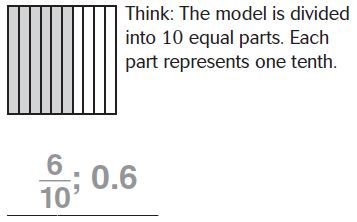Question 2.Type below: _________ Answer: 1 2/10 Explanation: The model is divided into 10 equal parts. Each part represents one-tenth. 1 2/10 is 1 whole and 2 tenths. Question 3.Type below: _________ Answer: 2 3/10 = 2.3 Explanation:Lesson 9.3 Write the number as hundredths in fraction form and decimal form. Question 4. $$\frac{8}{10}$$ Type below: _________ Answer: 80/1000.8 Explanation: Write 8/10 as an equivalent fraction. 8/10 =8×10/10×10 = 80/100 8 tenths is the same as 8 tenths 0 hundredths. So the decimal form = 0.8 Question 5. 0.1 Type below: _________ Answer: 50/1000.50 Explanation: Write 0.1 = 1/10 as an equivalent fraction. 1/10 =1×10/10×10 = 10/100 1 tenth is the same as 1 tenth 0 hundredths and also 0.1 Question 6. $$\frac{3}{10}$$ Type below: _________ Answer:Write 0.1 = 1/10 as an equivalent fraction. 3/10 =3×10/10×10 = 30/100 3 tenth is the same as 3 tenth 0 hundredths and also 0.3 Write the number as tenths in fraction form and decimal form. Question 7. $$\frac{60}{100}$$ Type below: _________ Answer: 6/10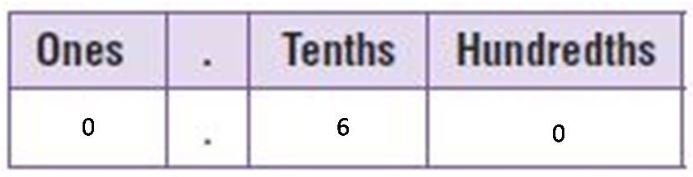0.6 Explanation: 10 is a common factor of the numerator and the denominator. 60/100 = 60÷10/100÷10 = 6/10 0.6 Thus the decimal form of the fraction $$\frac{60}{100}$$ is 0.6 Question 8. $$\frac{70}{100}$$ Type below: _________ Answer:Explanation: 10 is a common factor of the numerator and the denominator. 70/100 = 70÷10/100÷10 = 7/10 0.7 Thus the decimal form of the fraction $$\frac{70}{100}$$ is 0.7 Question 9. 0.20 Type below: _________ Answer: $$\frac{20}{100}$$ Explanation: The fraction form of 0.20 is $$\frac{20}{100}$$ Lesson 9.4 Write as a money amount and as a decimal in terms of dollars. Question 10. $$\frac{30}{100}$$ Dollars:$ _____ Decimal: _____

amount: $0.3 decimal: 0.3 of a dollar Explanation: 30/100 = 0.3 Thus the decimal form of the fraction $$\frac{30}{100}$$ is 0.3 Question 11. $$\frac{91}{100}$$ Dollars:$ _____ Decimal: _____

amount: $0.91 decimal: 0.91 of a dollar Explanation: 91/100 = 0.91 Thus the decimal form of the fraction $$\frac{91}{100}$$ is 0.91 Question 12. $$\frac{5}{100}$$ Dollars:$ _____ Decimal: _____

amount: $0.05 decimal: 0.05 of a dollar Explanation: 5/100 = 0.05 Thus the decimal form of the fraction $$\frac{5}{100}$$ is 0.05 Write the total money amount. Then write the amount as a fraction and as a decimal in terms of dollars. Question 13. 4 dimes, 9 pennies Answer: money amount:$0.49; fraction: 49/100; decimal: 0.49

Explanation:
Given that 4 dimes 9 pennies = (4 x 10/100) + (9 x 1/100) = 40/100 + 9/100 = 49/100

Question 14.
3 quarters, 1 dime

money amount: $0.85; fraction: 85/100; decimal: 0.85 Explanation: Given that 3 quarters 1 dime = (3 x 25/100) + (1 x 10/100) = 75/100 + 10/100 = 85/100 Question 15. 7 nickels, 2 pennies Answer: money amount:$0.37; fraction: 37100; decimal: 0.37

Explanation:
Given that 7 nickels 2 pennies = (7 x 5/100) + (2 x 1/100) = 35/100 + 2/100 = 37/100

### Common Core – Relate Fractions and Decimals – Page No. 186

Lesson 9.5

Question 1.
Camila, Jocelyn, and Audrey each earned $2.55. How much did the three girls earn altogether?$ _____

Answer: $7.65 Explanation: Given Camila, Jocelyn, and Audrey each earned$2.55
so Multiply $2.55 with 3 = 3 ×$2.55
we get three girls to earn altogether is $7.65 Question 2. Elijah, Xavier, and Adrian earned a total of$8.34. The boys shared the earnings equally. How much did each boy get?
$_____ Answer:$2.78

Explanation:
Given Elijah, Xavier, and Adrian earned a total of $8.34 so divide the total of$8.34 by 3 = 8.34/3
then we get the boys shared the earnings equally is $2.78 Question 3. Anthony saves$7 each week. In how many weeks will he have saved at least $40? _____ weeks Answer: 6 weeks Explanation: Given that, Anthony saves$7 each week.
We have to find how many weeks will he have saved at least $40$40/$7 = 6 (approx). Thus it takes 6 weeks to save at least$40.

Question 4.
Brianna has $2 less than Victoria. Victoria has$11 more than Damian. Damian has $6. How much money do they have in all?$ _____

Answer: $38 Explanation: Given, Brianna has$2 less than Victoria. Victoria has $11 more than Damian. This means that Victoria has 11 more than Damian, and since Damian has 6, Victoria has 17. Plug this into the fact that Brianna has 2 less than Victoria, or 15, to get 6 + 17 + 15 = 38 dollars. Lesson 9.6 Find the sum. Question 5. $$\frac{6}{10}+\frac{39}{100}$$ $$\frac{□}{□}$$ Answer: 99/100 Explanation: 6/10+39/100. Write the addends as fractions with a common denominator 6/10 = 6X10/10X10 = 60/100. 60/100+39/100 = 99/100 Question 6. $$\frac{14}{100}+\frac{8}{10}$$ $$\frac{□}{□}$$ Answer: 94/100 Explanation: 14/100+8/10. Write the addends as fractions with a common denominator 8/10 = 8X10/10X10 = 80/100. 14/100+80/100 = 94/100. Question 7. $$\frac{4}{10}+\frac{18}{100}$$ $$\frac{□}{□}$$ Answer: 58/100 Explanation: 4/10+18/100. Write the addends as fractions with a common denominator 4/10 = 4X10/10X10 = 40/100. 18/100+40/100 = 58/100 Question 8. $$\frac{5}{10}+\frac{16}{100}$$ $$\frac{□}{□}$$ Answer: 58/100 Explanation: 5/10+16/100. Write the addends as fractions with a common denominator 5/10 = 5X10/10X10 = 50/100. 16/100+50/100 = 66/100 Question 9.$0.43 + $0.20$ _____

$0.63 Explanation: Think 0.43 as 43 hundredths = 43/100. Think 0.20 as 20 hundredths = 20/100. Write the addends as fractions with a common denominator 43/100 + 20/100 = 63/100 = 0.63 Question 10.$0.07 + $0.35$ _____

$0.42 Explanation: Think 0.07 as 07 hundredths = 7/100. Think 0.35 as 35 hundredths = 35/100. Write the addends as fractions with a common denominator 7/100 + 35/100 = 42/100 = 0.42 Question 11.$0.80 + $0.15 =$ _____

$0.95 Explanation: Think 0.80 as 80 hundredths = 80/100. Think 0.15 as 15 hundredths = 15/100. Write the addends as fractions with a common denominator 80/100 + 15/100 = 95/100 = 0.95 Question 12.$0.52 + $0.28$ _____

\$0.80

Explanation:
Think 0.52 as 52 hundredths = 52/100.
Think 0.28 as 28 hundredths = 28/100.
Write the addends as fractions with a common denominator
52/100 + 28/100 = 80/100 = 0.80

Lesson 9.7

Compare. Write<, >, or =.

Question 13.
0.3 _____ 0.39

0.3 < 0.39.

Explanation:
0.3 is 3 tenths, which is equivalent to 30 hundredths.
0.39 is 39 hundredths.
30 hundredths < 39 hundredths. So, 0.3 < 0.39.

Question 14.
0.9 _____ 0.90

0.9 = 0.90

Explanation:
0.9 is 9 tenths, which is equivalent to 90 hundredths.
0.90 is 90 hundredths.
90 hundredths = 90 hundredths. So, 0.9 = 0.90.

Question 15.
0.54 _____ 0.45

0.54 > 0.45

Explanation:
0.54 is 5.4 tenths, which is equivalent to 54 hundredths.
0.45 is 45 hundredths.
54 hundredths > 45 hundredths. So, 0.54 > 0.45.

Question 16.
0.04 _____ 0.06

0.04 < 0.06

Explanation:
0.04 is 0.4 tenths, which is equivalent to 4 hundredths.
0.06 is 0.6 hundredths.
0.4 hundredths < 0.6 hundredths. So, 0.04 < 0.06

Question 17.
0.7 _____ 0.70

0.7 = 0.70

Explanation:
0.7 is 7 tenths, which is equivalent to 70 hundredths.
0.70 is 70 hundredths.
70 hundredths = 70 hundredths. So, 0.7 = 0.70.

Question 18.
0.36 _____ 0.51

0.36 < 0.51.

Explanation:
0.36 is 3.6 tenths, which is equivalent to 36 hundredths.
0.51 is 51 hundredths.
36 hundredths < 51 hundredths. So, 0.36 < 0.51.

Question 19.
0.8 _____ 0.67

0.8 > 0.67.

Explanation:
0.8 is 8.0 tenths, which is equivalent to 80 hundredths.
0.67 is 67 hundredths.
80 hundredths > 67 hundredths. So, 0.80 > 0.67.

Question 20.
0.63 _____ 0.48

0.63 > 0.48.

Explanation:
0.63 is 6.3 tenths, which is equivalent to 63 hundredths.
0.48 is 48 hundredths.
63 hundredths > 48 hundredths. So,0.63 > 0.48.

Compare. Write true or false.

Question 21.
0.32 > 0.23
_____

True

Explanation:
0.32 is Greater than 0.23 and the left side of the number line. So, 0.32 < 0.23. The answer is True.

Question 22.
0.86 = 0.9
_____

false

Explanation:
86 tenths is less than 90 tenths. So, 0.86 < 0.9. So, the answer is false.

Question 23.
0.68 < 0.83
_____

true

Explanation:
6 tenths is less than 8 tenths. So, 0.68 < 0.83. So, the answer is true.

Question 24.
0.97 > 0.94
_____